# 我画了20张图，终于让女朋友学会了翻转链表

danielmou

0收藏

### 前言

1. 什么是链表，链表的优缺点
2. 链表的表示及基本操作
3. 面试中链表的常见解题思路---翻转

### 什么是链表

#### 链表的定义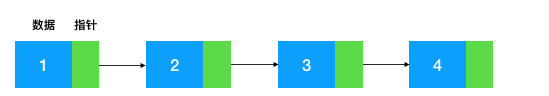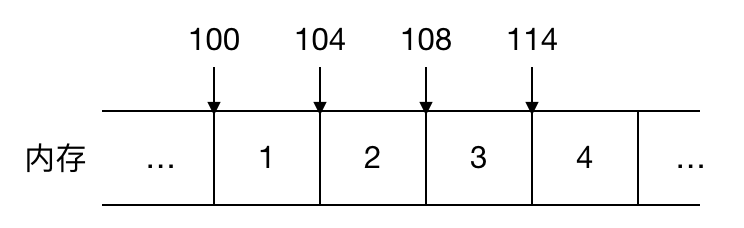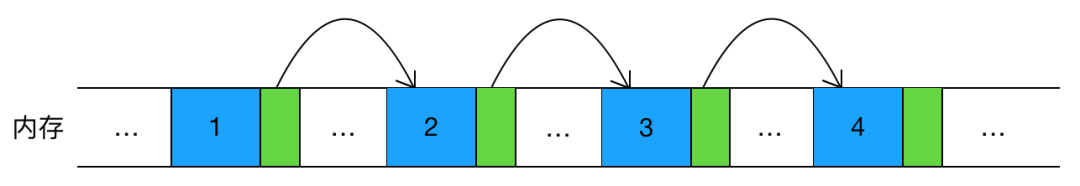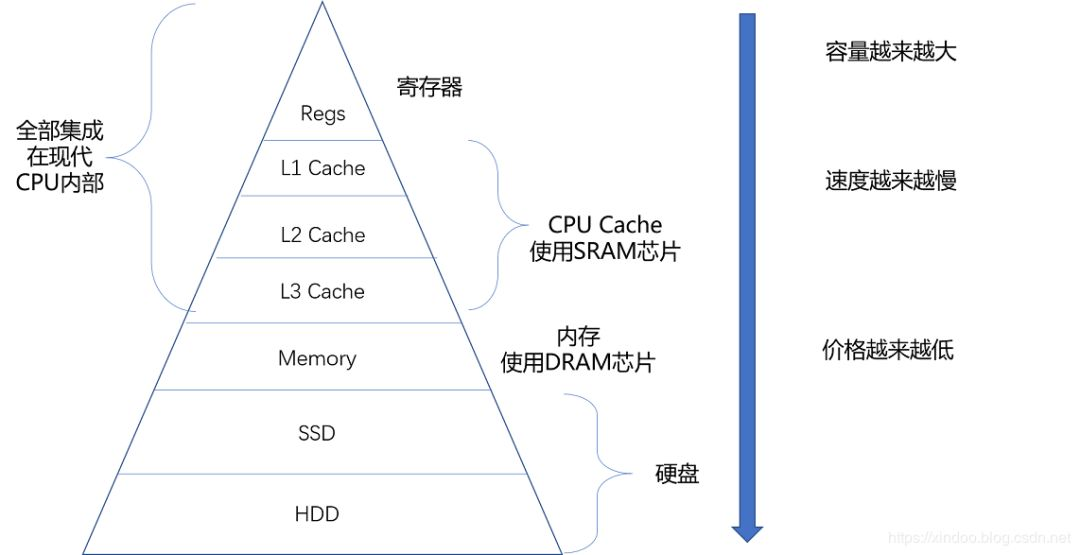• 大内存空间分配

• 元素频繁删除和插入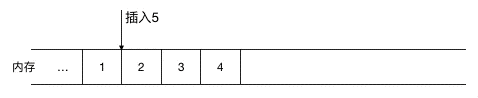(在 1，2 间插入 5，需要把2，3，4 同时往后移一位)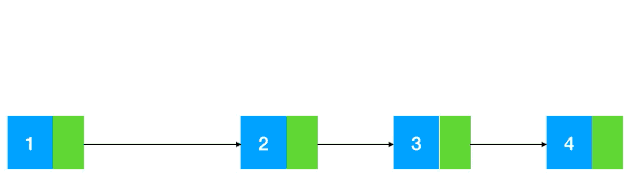### 链表的表示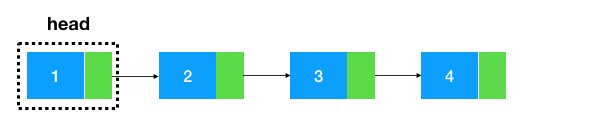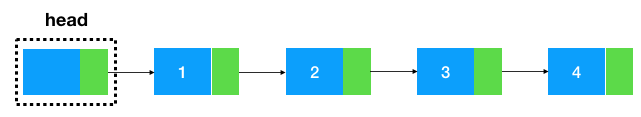``````/**
* 链表中的结点，data代表节点的值，next是指向下一个节点的引用
*/
class Node {
int data;// 结点的数组域，值
Node next =     null;// 节点的引用，指向下一个节点
public Node(    int{
this.data = data;
}
}

/**
* 链表
*/
int length = 0; // 链表长度，非必须，可不加
Node head =     null; // 头结点

}     else {
while (tmp.next !=     null) {
tmp.next = tmp;
}
tmp.next =     new Node(val);
}
}
}``````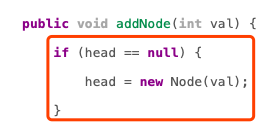1. 每插入一个元素都要对头结点进行判空比较，如果一个链表有很多元素需要插入，就需要进行很多次的判空处理，不是那么高效
2. 头结点与其他结点插入逻辑不统一（一个需要判空后再插入，一个不需要判空直接插入）,从程序逻辑性来说不是那么合理（因为结点与结点是平级，添加逻辑理应相同）

``````    public     class LinkedList {
int length = 0; // 链表长度，非必须，可不加
Node head =     new Node(0); // 哨兵结点
while (tmp.next !=     null) {
tmp.next = tmp;
}
tmp.next =     new Node(val);
}
}``````

### 链表常见解题套路

#### 热身赛``````    public     class LinkedList {
int length = 0; // 链表长度，非必须，可不加
Node head =     new Node(0); // 哨兵节点

// 头插法
// 1.构造新结点
Node newNode =     new Node(val);
// 2.新结点指向头结点之后的结点
// 3.头结点指向新结点
}

public     static     void main(String[] args){
int[] arr = {1,2,3,4};
// 头插法构造链表
for (    int i = 0; i < arr.length; i++) {
}
// 打印链表,将打印 4-->3-->2-->1
}
}``````

#### 小试牛刀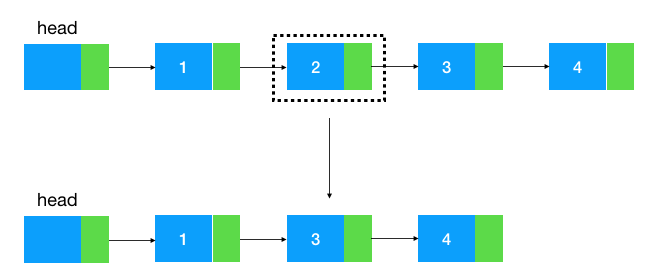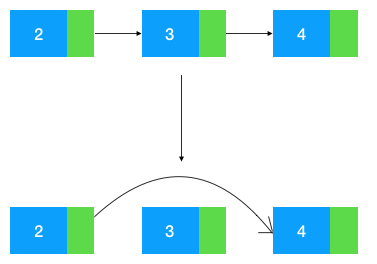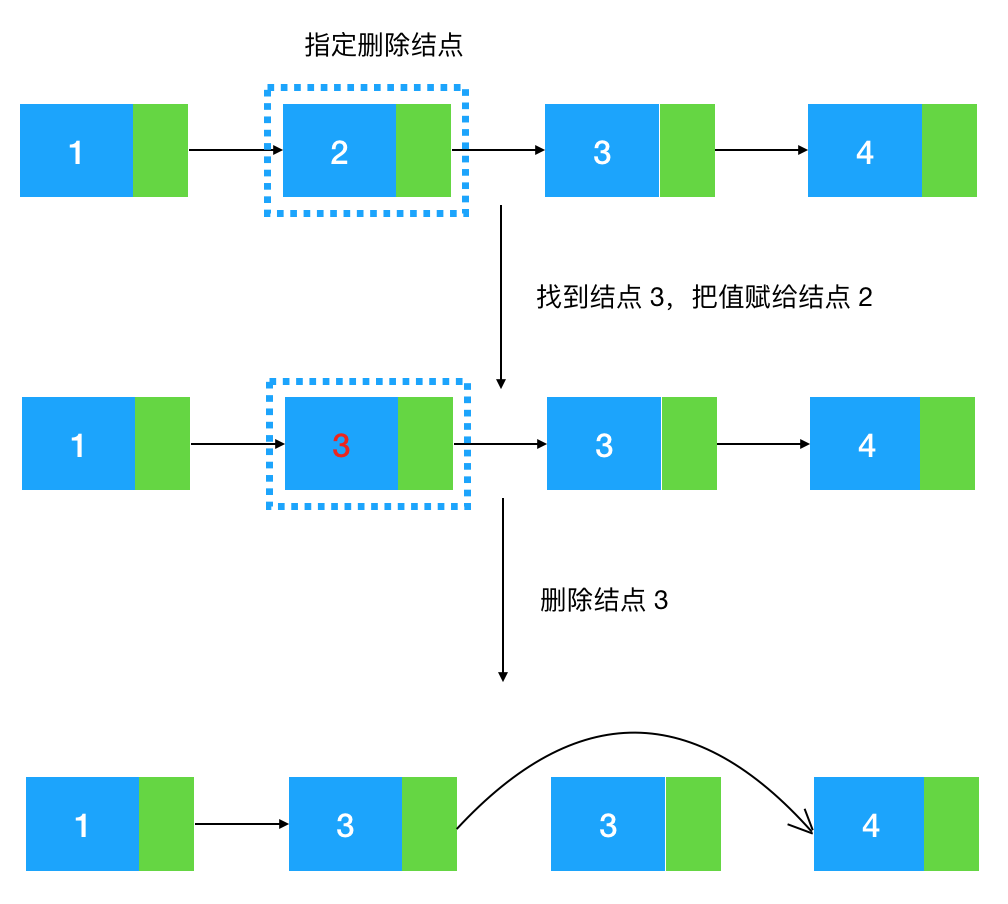``````/**
* 删除指定的结点
* @param deletedNode
*/
public     void removeSelectedNode(Node deletedNode){
// 如果此结点是尾结点我们还是要从头遍历到尾结点的前继结点，再将尾结点删除
if (deletedNode.next ==     null) {
while (tmp.next != deletedNode) {
tmp = tmp.next;
}
// 找到尾结点的前继结点，把尾结点删除
tmp.next =     null;
}     else {
Node nextNode = deletedNode.next;
// 将删除结点的后继结点的值赋给被删除结点
deletedNode.data = nextNode.data;
// 将 nextNode 结点删除
deletedNode.next = nextNode.next;
nextNode.next =     null;
}
}``````

#### 入门到进阶:链表翻转

• 递归翻转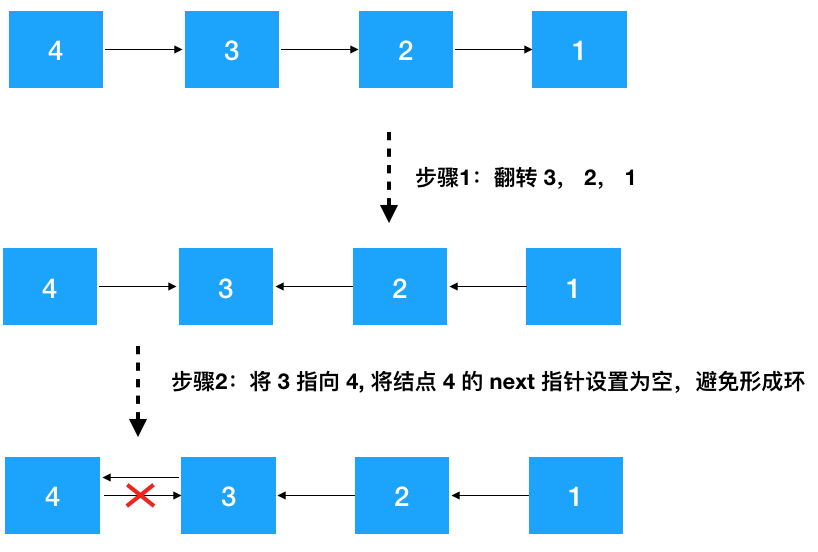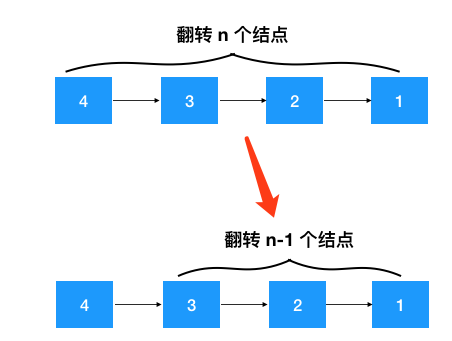1、定义递归函数,明确函数的功能 根据以上分析，这个递归函数的功能显然是翻转某个节点开始的链表，然后返回新的头结点

``````/**
* 翻转结点 node 开始的链表
*/
}``````

2、寻找递推公式 上文中已经详细画出了翻转链表的步骤，简单总结一下递推步骤如下

• 针对结点 node (值为 4), 先翻转 node 之后的结点   invert(node->next) ,翻转之后 4--->3--->2--->1变成了 4--->3<---2<---1
• 再把 node 节点的下个节点（3）指向 node，node 的后继节点设置为空（避免形成环），此时变成了 4<---3<---2<---1
• 返回新的头结点，因为此时新的头节点从原来的 4 变成了 1，需要重新设置一下 head

``````/**
* 递归翻转结点 node 开始的链表
*/
if (node.next ==     null) {
return node;
}

// 步骤 1: 先翻转 node 之后的链表

// 步骤 2: 再把原 node 节点后继结点的后继结点指向 node，node 的后继节点设置为空(防止形成环)
node.next.next = node;
node.next =     null;

// 步骤 3: 返回翻转后的头结点
}

public     static     void main(String[] args){
int[] arr = {4,3,2,1};
for (    int i = 0; i < arr.length; i++) {
}
// 翻转后别忘了设置头结点的后继结点！
}``````

4、计算时间/空间复杂度 由于递归调用了 n 次 invertLinkedList 函数，所以时间复杂度显然是 O(n), 空间复杂度呢，没有用到额外的空间，但是由于递归调用了  n 次 invertLinkedList 函数，压了 n 次栈，所以空间复杂度也是 O(n)。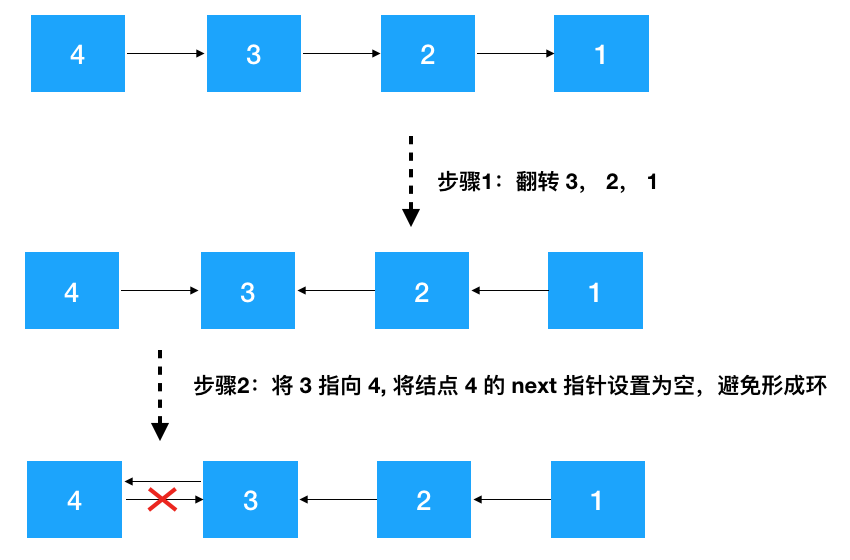• 非递归翻转链表（迭代解法）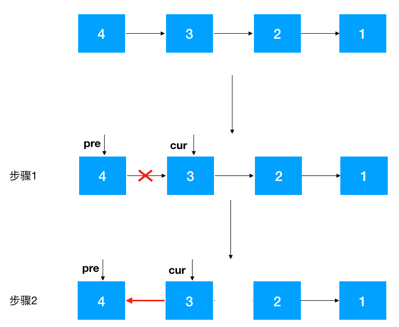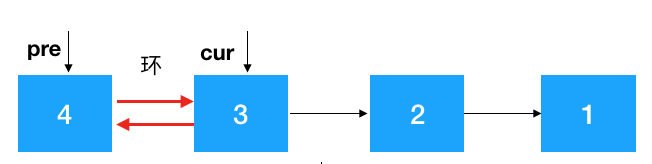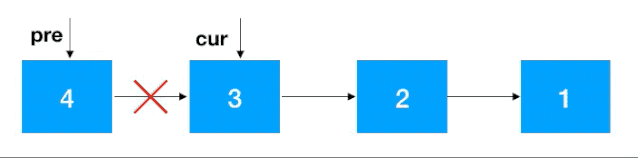``````/**
* 迭代翻转
*/
// 步骤 1
Node cur = pre.getNext();
pre.setNext(    null);   // pre 是头结点，避免翻转链表后形成环

// 步骤 2
while (cur !=     null) {
/**
* 务必注意!!!：在 cur 指向 pre 之前一定要先保留 cur 的后继结点，不然如果 cur 先指向 pre，之后就再也找不到后继结点了
*/
Node next = cur.getNext();
cur.setNext(pre);
pre = cur;
cur = next;
}
// 此时 pre 指向的是原链表的尾结点，翻转后即为链表 head 的后继结点
}``````

1. 根据 from 和 to 找到 from-1, from, to,  to+1 四个结点(注意临界条件，如果 from 从头结点开始，则 from-1 结点为空, 翻转后需要把 to 设置为头结点的后继结点， from 和 to 结点也可能超过尾结点,这两种情况不符合条件不翻转)。
2. 对 from 到 to 的结点进行翻转
3. 将 from-1 节点指向 to 结点，将 from 结点指向 to + 1 结点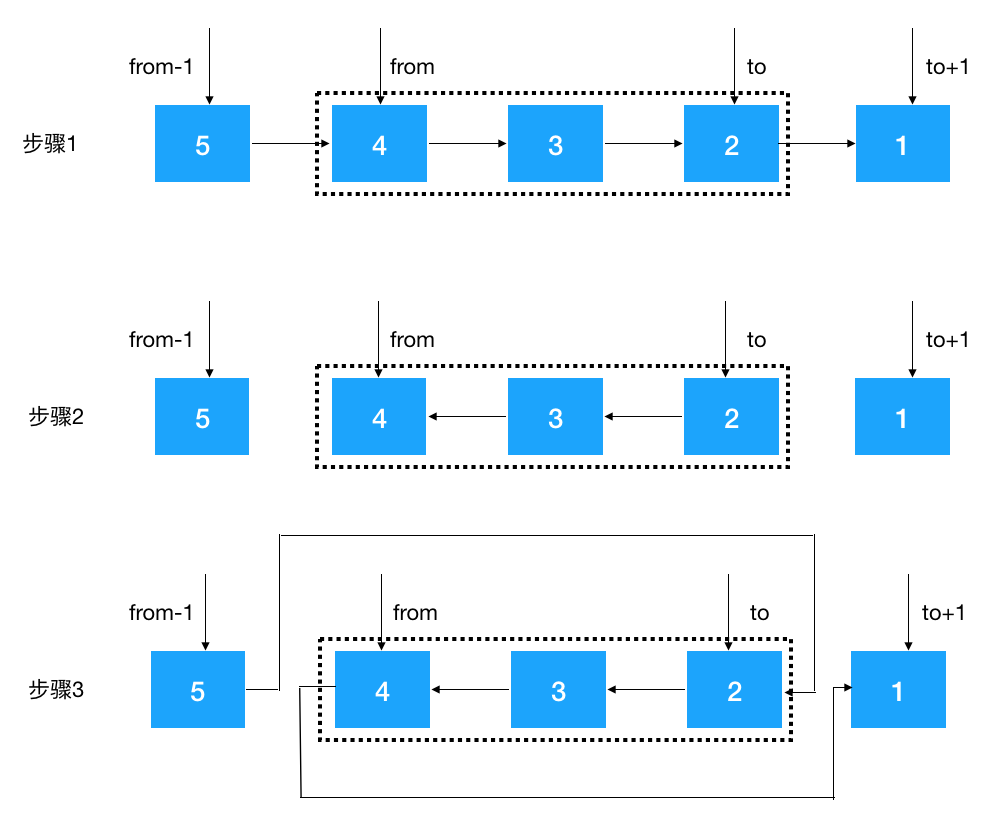``````/**
* 迭代翻转 from 到 to 的结点
*/
public     void iterationInvertLinkedList(    int fromIndex,     int     throws{

Node fromPre =     null;            // from-1结点
Node from =     null;               // from 结点
Node to =     null;                 // to 结点
Node toNext =     null;             // to+1 结点

// 步骤 1：找到  from-1, from, to,  to+1 这四个结点
int curIndex = 1;      // 头结点的index为1
while (tmp !=     null) {
if (curIndex == fromIndex-1) {
fromPre = tmp;
}     else     if (curIndex == fromIndex) {
from = tmp;
}     else     if (curIndex == toIndex) {
to = tmp;
}     else     if (curIndex == toIndex+1) {
toNext = tmp;
}
tmp = tmp.next;
curIndex++;
}

if (from ==     null || to ==     null) {
// from 或 to 都超过尾结点不翻转
throw     new Exception("不符合条件");
}

// 步骤2：以下使用循环迭代法翻转从 from 到 to 的结点
Node pre = from;
Node cur = pre.next;
while (cur != toNext) {
Node next = cur.next;
cur.next = pre;
pre = cur;
cur = next;
}

// 步骤3：将 from-1 节点指向 to 结点（如果从 head 的后继结点开始翻转，则需要重新设置 head 的后继结点），将 from 结点指向 to + 1 结点
if (fromPre !=     null) {
fromPre.next = to;
}     else {
}
from.next = toNext;
}``````

• 你的算法只能使用常数的额外空间。
• 你不能只是单纯的改变节点内部的值，而是需要实际的进行节点交换。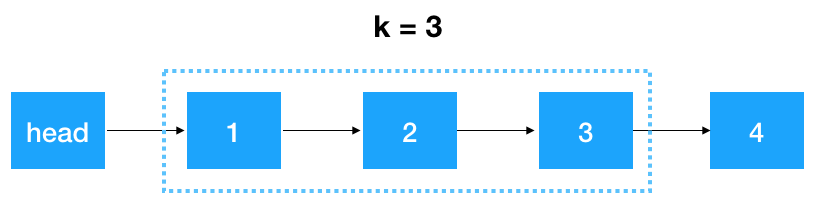• 首先，我们要记录 3 个一组这一段链表的前继结点,定义为 startKPre,然后再定义一个 step, 从这一段的头结点 (1）开始遍历 2 次，找出这段链表的起始和终止结点，如下图示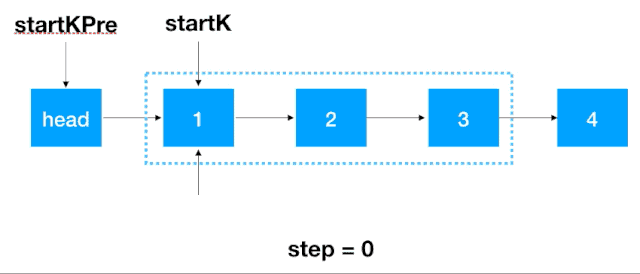• 找到 startK 和 endK 之后，根据之前的迭代翻转法对 startK 和 endK 的这段链表进行翻转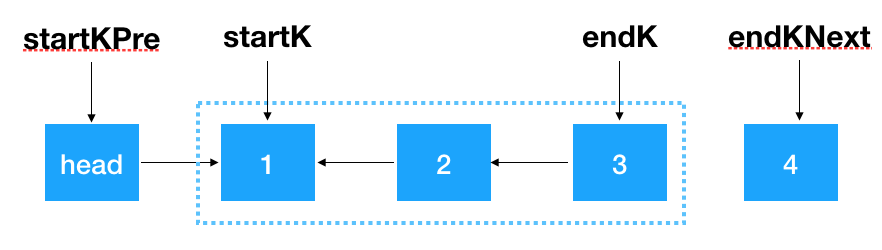• 然后将 startKPre 指向 endK,将 startK 指向 endKNext，即完成了对 k 个一组结点的翻转。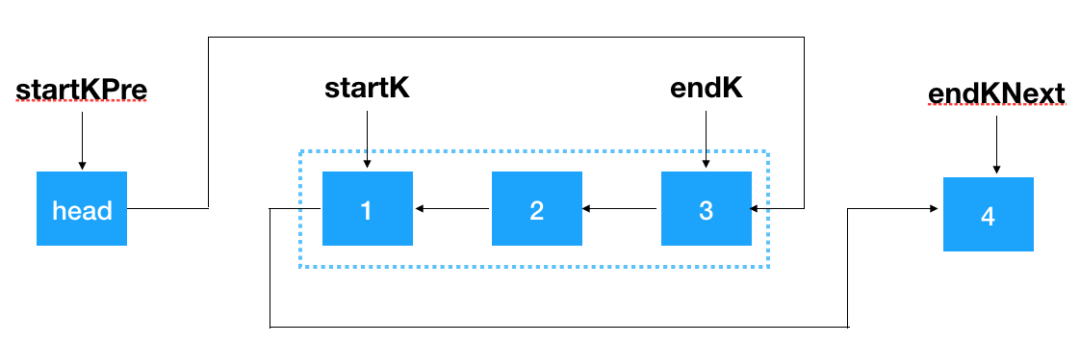``````/**
* 每 k 个一组翻转链表
* @param k
*/
int step = 0;               // 计数，用来找出首结点和尾结点

Node startK =     null;         // k个一组链表中的头结点
Node startKPre = head;      // k个一组链表头结点的前置结点
Node endK;                  // k个一组链表中的尾结点
while (tmp !=     null) {
// tmp 的下一个节点,因为由于翻转，tmp 的后继结点会变,要提前保存
Node tmpNext = tmp.next;
if (step == 0) {
// k 个一组链表区间的头结点
startK = tmp;
step++;
}     else     if (step == k-1) {
// 此时找到了 k 个一组链表区间的尾结点（endK）,对这段链表用迭代进行翻转
endK = tmp;
Node pre = startK;
Node cur = startK.next;
if (cur ==     null) {
break;
}
Node endKNext = endK.next;
while (cur != endKNext) {
Node next = cur.next;
cur.next = pre;
pre = cur;
cur = next;
}
// 翻转后此时 endK 和 startK 分别是是 k 个一组链表中的首尾结点
startKPre.next = endK;
startK.next = endKNext;

// 当前的 k 个一组翻转完了，开始下一个 k 个一组的翻转
startKPre = startK;
step = 0;
}     else {
step++;
}
tmp = tmpNext;
}
}``````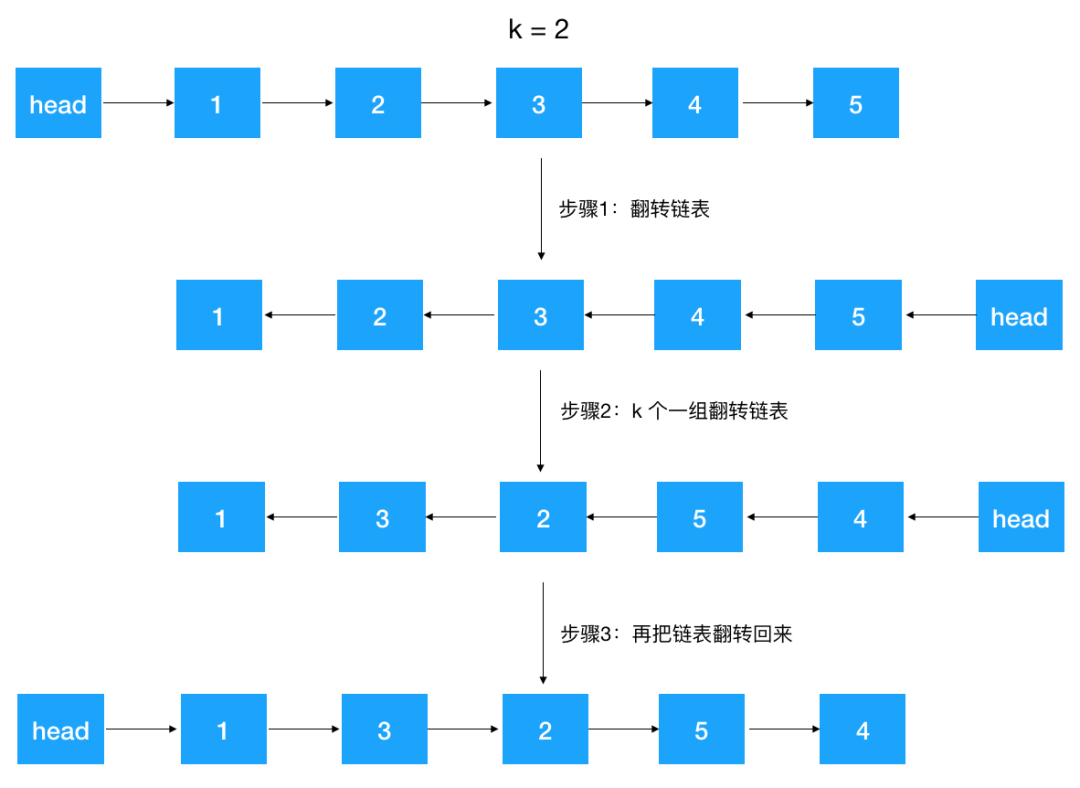``````/**
* 逆序每 k 个一组翻转链表
* @param k
*/
// 先翻转链表
// k 个一组翻转链表
// 再次翻转链表
}``````

### 总结帖子
视频
声望
粉丝
社区精华内容